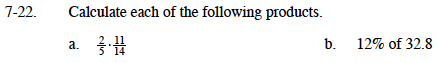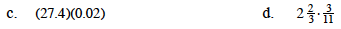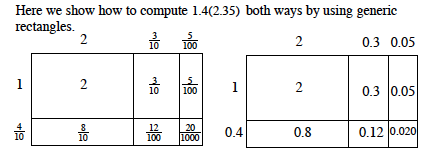### Home > MC1 > Chapter 7 > Lesson 7.1.2 > Problem7-22

7-22.$\frac{2}{5}\cdot\frac{11}{14}= \frac{11}{35}$

0.12(32.8) = ?Try using a generic rectangle like theMath Notes box from Lesson 6.3.1 pictured below.

This can be written as:

$\left ( \frac{8}{3} \right )\left ( \frac{3}{11} \right )$